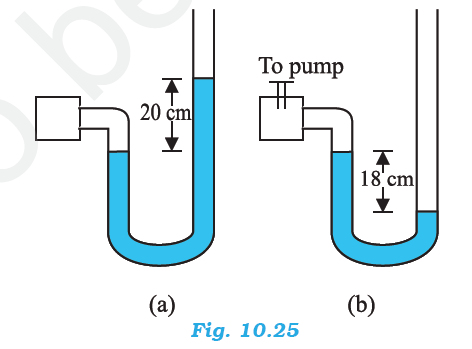## Pages

### Mechanical Properties of Fluids NCERT Solutions Class 11 Physics - Solved Exercise Question 10.22

Question 10.22:
A manometer reads the pressure of a gas in an enclosure as shown in Fig. 10.25 (a) When a pump removes some of the gas, the manometer reads as in Fig. 10.25 (b) The liquid used in the manometers is mercury and the atmospheric pressure is 76 cm of mercury.
(a) Give the absolute and gauge pressure of the gas in the enclosure for cases (a) and (b), in units of cm of mercury.
(b) How would the levels change in case (b) if 13.6 cm of water (immiscible with mercury) are poured into the right limb of the manometer? (Ignore the small change in the volume of the gas).Solution:
(a) For figure (a)
Atmospheric pressure, P0 = 76 cm of Hg
Difference between the levels of mercury in the two limbs gives gauge pressure
Hence, gauge pressure is 20 cm of Hg.
Absolute pressure = Atmospheric pressure + Gauge pressure
= 76 + 20 = 96 cm of Hg

For figure (b)
Difference between the levels of mercury in the two limbs = –18 cm
Hence, gauge pressure is –18 cm of Hg.
Absolute pressure = Atmospheric pressure + Gauge pressure
= 76 cm – 18 cm = 58 cm

(b) 13.6 cm of water is poured into the right limb of figure (b).
Relative density of mercury = 13.6
Hence, a column of 13.6 cm of water is equivalent to 1 cm of mercury.
Let h be the difference between the levels of mercury in the two limbs.
The pressure in the right limb is given as:
PR = Atmospheric pressure + 1 cm of Hg
= 76 + 1 = 77 cm of Hg … (i)
The mercury column will rise in the left limb.
Hence, pressure in the left limb, PL = 58 + h               .....(ii)
Equating equations (i) and (ii), we get:
77 = 58 + h
h = 19 cm
Hence, the difference between the levels of mercury in the two limbs will be 19 cm.

#### 1 comment:

1.I got what you mean , a debt of gratitude is in order for posting .Woh I am cheerful to discover this site through google. socket screws